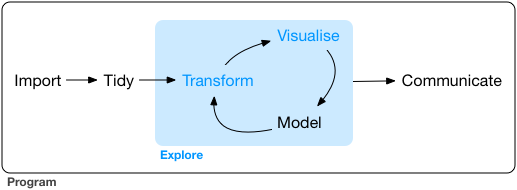# R for Data Science Day 1

I am incredibly excited that RStudio has begun an instructor certification program based on the Carpentries, so of course I signed up as soon as my overcommited nature allowed! This also provides me with the excuse and motivation to finally formally work my way through R for Data Science, a book I have read while waiting for GTT tests during my pregnancy and google-landed upon an umpteen number of times while debugging code, but never taken the time to sit down and do the exercises for - and of course the pedagogue in me knows quite well that THAT is how you actually learn and internalise the principles and concepts in any material, especially if it deals with programming and analysis. So over the next few weeks I plan to work my way through R4DS, and this post is the first in which I dive into the exercises.

## Personal, highly non-exhaustive notes on section I: Explore

``library(tidyverse)``

### Steps of the data pipeline:• Import: take data stored in a file, database, or web API, and load it into a data frame in R.

### Wrangling:

• Tidying - storing data in a consistent form that matches the semantics of the dataset with the way it is stored. In brief, when your data is tidy, each column is a variable, and each row is an observation.

• Transformation
• narrowing in on observations of interest (like all people in one city, or all data from the last year),
• creating new variables that are functions of existing variables (like computing speed from distance and time),
• calculating a set of summary statistics (like counts or means). Together, tidying and transforming are called wrangling

## Small data vs big data

• Small/medium data: hundreds of megabytes of data, and with a little care up to 1-2 Gb of data.
• If you’re routinely working with larger data (10-100 Gb, say), you should learn more about data.table.

## Is big data really big? Two ways of thinking small about big data

### Sampling

Sampling may be enough to answer the question.

### Your big data problem is actually a large number of small data problems

• Each individual problem might fit in memory, but you have millions of them. For example, you might want to fit a model to each person in your dataset. That would be trivial if you had just 10 or 100 people, but instead you have a million.
• So you need a system (like Hadoop or Spark) that allows you to send different datasets to different computers for processing.
• Once you’ve figured out how to answer the question for a single subset using the tools described in this book, you can use tools like sparklyr, rhipe, and ddr to solve it for the full dataset.

### New (to me) `ggplot()` aesthetics

• `stroke` - is either the size of the point (for a default `geom_point()`) OR, if used with shape 21-25, which have both a colour and a fill, is the thickness of the stroke around the plotted shape.

• You can generally use geoms and stats interchangeably! For example, you can use stat_count() instead of geom_bar() to make the same plot!

``ggplot(data = diamonds) + geom_bar(aes(x = cut, y = ..count.. / sum(..count..), fill = color))````````# not really new, but I'm sure I'll forget position = "fill"
ggplot(data = diamonds) +
geom_bar(mapping = aes(x = cut, fill = clarity), position = "fill")````````````# pie chart from bar
ggplot(data = diamonds) +
geom_bar(mapping = aes(x = 1, fill = clarity)) + coord_polar(theta = "y")``````On average, humans are best able to perceive differences in angles relative to 45 degrees. The function ggthemes::bank_slopes() will calculate the optimal aspect ratio to bank slopes to 45-degrees.

### Very clear table of ggplot mappings (from here)

geom default stat shared docs
geom_abline()
geom_hline()
geom_vline()
geom_bar() stat_count() x
geom_col()
geom_bin2d() stat_bin_2d() x
geom_blank()
geom_boxplot() stat_boxplot() x
geom_countour() stat_countour() x
geom_count() stat_sum() x
geom_density() stat_density() x
geom_density_2d() stat_density_2d() x
geom_dotplot()
geom_errorbarh()
geom_hex() stat_hex() x
geom_freqpoly() stat_bin() x
geom_histogram() stat_bin() x
geom_crossbar()
geom_errorbar()
geom_linerange()
geom_pointrange()
geom_map()
geom_point()
geom_map()
geom_path()
geom_line()
geom_step()
geom_point()
geom_polygon()
geom_qq_line() stat_qq_line() x
geom_qq() stat_qq() x
geom_quantile() stat_quantile() x
geom_ribbon()
geom_area()
geom_rug()
geom_smooth() stat_smooth() x
geom_spoke()
geom_label()
geom_text()
geom_raster()
geom_rect()
geom_tile()
geom_violin() stat_ydensity() x
geom_sf() stat_sf() x

### Very clear table of ggplot stats (from here)

stat default geom shared docs
stat_ecdf() geom_step()
stat_ellipse() geom_path()
stat_function() geom_path()
stat_identity() geom_point()
stat_summary_2d() geom_tile()
stat_summary_hex() geom_hex()
stat_summary_bin() geom_pointrange()
stat_summary() geom_pointrange()
stat_unique() geom_point()
stat_count() geom_bar() x
stat_bin_2d() geom_tile() x
stat_boxplot() geom_boxplot() x
stat_countour() geom_contour() x
stat_sum() geom_point() x
stat_density() geom_area() x
stat_density_2d() geom_density_2d() x
stat_bin_hex() geom_hex() x
stat_bin() geom_bar() x
stat_qq_line() geom_path() x
stat_qq() geom_point() x
stat_quantile() geom_quantile() x
stat_smooth() geom_smooth() x
stat_ydensity() geom_violin() x
stat_sf() geom_rect() x